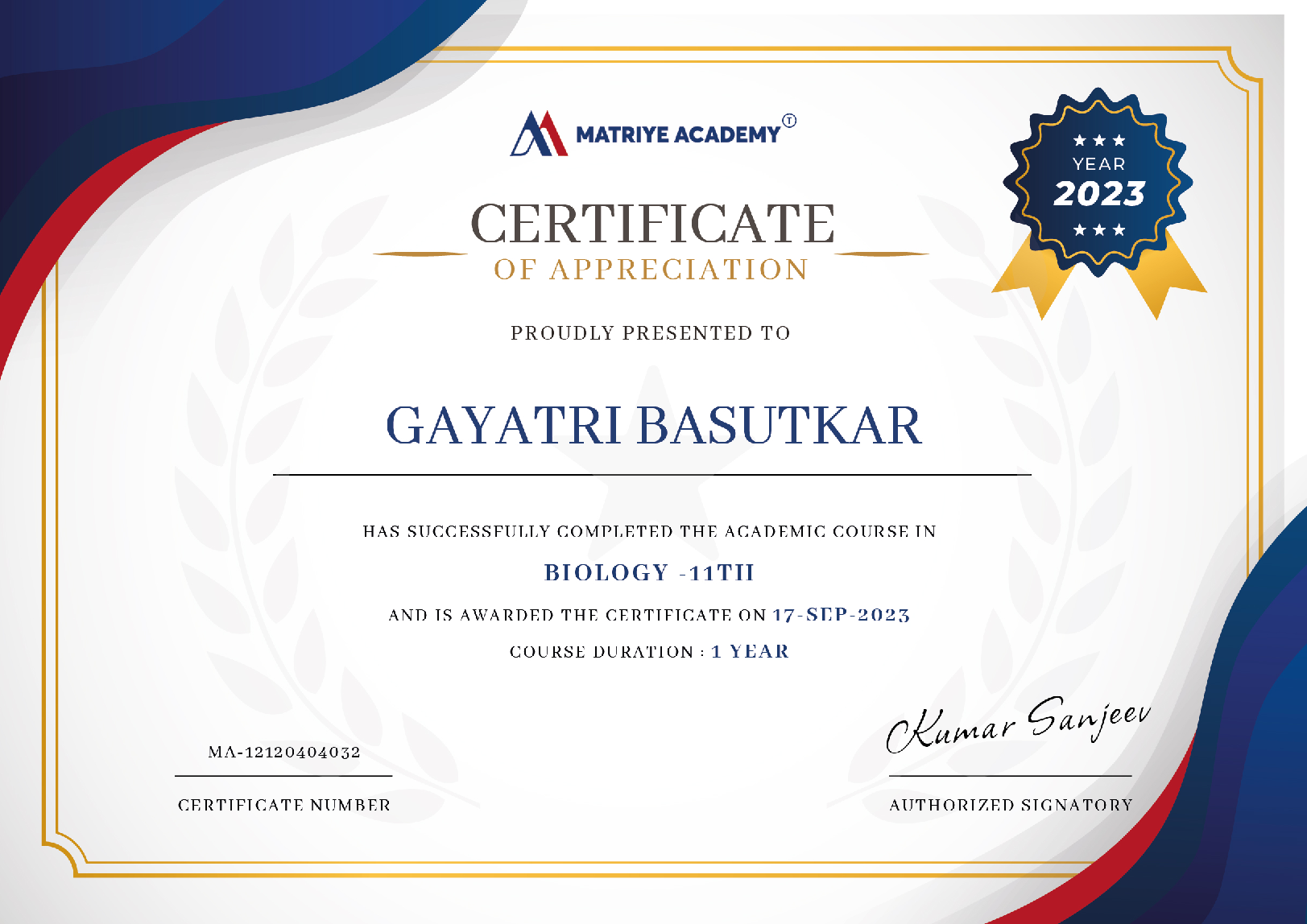# Maths

CBSE 9TH

Students in Class 9 Math may derive proofs for mathematical concepts involving parallel lines, triangles, quadrilaterals, circles, and so on.##### Runali Deb Roy

Last Update : 08 Feb 2023

(4)

## Overview of CBSE 9th Grade Mathematics Curriculum

The entire academic year has been split by the board. Furthermore, the board has established test patterns for both terms. Through the CBSE Test Pattern, students may learn about many important exam materials. Students may learn about the test time, marks, and types of questions here.

Our website contains comprehensive information about CBSE Class 9 Maths, including example papers, NCERT Solutions, books, curriculum, exam patterns, and more. They can go through everything in this post and prepare for the examinations accordingly. Continue reading to learn more about CBSE Class 9 Maths.

### Topics Covered in CBSE 9th Grade Mathematics Course

• Students can use logical reasoning to classify real numbers, prove their qualities, and apply them in various contexts.
• Recognize or categorize polynomials in algebraic expressions and factor them using algebraic identities.
• The concepts studied in class 9 Maths constitute the cornerstone of higher education, pupils will perform well in subsequent classes.
• Students can detect or categorize polynomials among algebraic expressions and factorizes them by using relevant algebraic identities.

15 Lessons 45m

### 2023 CBSE Class 9 Maths Exam Pattern

The CBSE has issued a specific evaluation methodology for Class 9 exams. The full academic year has been split by the board. Furthermore, the board has established test patterns for both terms. Through the CBSE Test Pattern, students may learn about many important exam materials. Students may learn about the test time, marks, and types of questions here.

### Class 9 Math's

Class 9 Math is an exciting topic that serves as a watershed moment in the lives of many students. Understanding class 9 Math will help pupils perform well in higher classes because the concepts studied are the foundation of higher education.

Students can also go to the class 9 Math's index page to obtain full notes for each chapter's subtopics. Class 9 Math's notes are highly beneficial in helping pupils grasp ideas in depth. The following is a list of chapter-by-chapter notes for class 9 Math.

### Important of Class 9 Mathematics

Class 9 Mathematics sets the groundwork for higher classes since students take their first board exam in class 10. Mathematics must be practiced continuously from the start in order for the concepts to be clear. Students in class 9 require thorough preparation for the test since it serves as the foundation for the class 10 board exam. Good grades in class 9 boost a child's confidence and inspire them to do well in class 10.## 1

##### Unit I: Number Systems
5
4 ratings 2 reviews

1. Real Numbers (18 Periods)

1. Review of representation of natural numbers, integers, and rational numbers on the number line. Rational numbers as recurring/ terminating decimals. Operations on real numbers.
2. Examples of non-recurring/non-terminating decimals. Existence of non-rational numbers (irrational numbers) such as âˆš2, âˆš3 and their representation on the number line. Explaining that every real number is represented by a unique point on the number line and, conversely, viz., every point on the number line represents a unique real number.
3. Definition of nth root of a real number.
4. Rationalization (with precise meaning) of real numbers of the type (and their combinations) where x and y are natural numbers and a and b are integers.
5. Recall of laws of exponents with integral powers. Rational exponents with positive real bases (to be done by particular cases, allowing the learner to arrive at the general laws.)

## 2

##### Unit II: Algebra
5
4 ratings 4 reviews

1. Polynomials (26 Periods)

• Definition of a polynomial in one variable, with examples and counter examples. Coefficients of a polynomial, terms of a polynomial, and zero polynomial. Degree of a polynomial. Constant, linear, quadratic, and cubic polynomials. Monomials, binomials, trinomials. Factors and multiples. Zeros of a polynomial. Motivate and State the Remainder Theorem with examples. Statement and proof of the Factor Theorem. Factorization of ax2 + bx + c, a â‰  0 where a, b, and c are real numbers, and of cubic polynomials using the Factor Theorem. Recall algebraic expressions and identities. Verification of identities:

2. Linear Equations in Two Variables (16 Periods)

Recall linear equations in one variable. Introduction to the equation in two variables. Focus on linear equations of the type ax + by + c=0. Explain that a linear equation in two variables has infinitely many solutions and justify their being written as ordered pairs of real numbers, plotting them and showing that they lie on a line.

### Course Instructor##### Shruti Koli
(4)
25 Tutorials 100 Students

It's well-organized and simple to use. Lesson themes are fascinating, and genuine student writing samples will stimulate pupils. Some well-designed slideshows are included as supplemental content.##### Ravikant Sharma
(4)
25 Tutorials 100 Students

The instructor was extremely well-informed and eloquent. This was my first experience taking a lesson remotely, and I appreciated how, although being in a class with other people, the real physical location was private, peaceful, and conducive to studying!

### Start Learning Today

###### Financial aid available
• Taught by top companies and universities
• Affordable programs
• Apply your skills with hands-on projects
• Learn on your own schedule